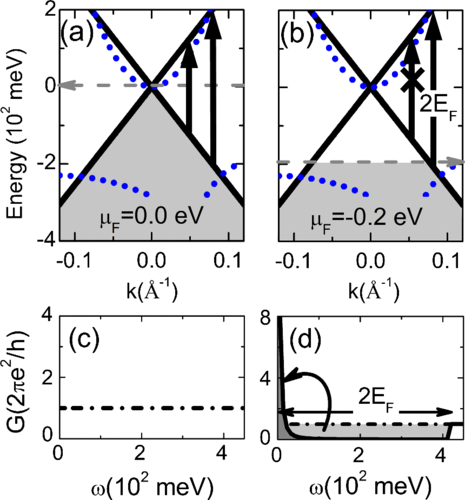# Sum-Rule Constraints on the Surface State Conductance of Topological Insulators### 摘要

We report the Drude oscillator strength $D$ and the magnitude of the bulk band gap $E_g$ of the epitaxially grown, topological insulator (Bi,Sb)$_2$Te$_3$. The magnitude of $E_g$, in conjunction with the model independent $f$-sum rule, allows us to establish an upper bound for the magnitude of $D$ expected in a typical Dirac-like system composed of linear bands. The experimentally observed $D$ is found to be at or below this theoretical upper bound, demonstrating the effectiveness of alloying in eliminating bulk charge carriers. Moreover, direct comparison of the measured $D$ to magnetoresistance measurements of the same sample supports assignment of the observed low-energy conduction to topological surface states.

In Physical Review Letters